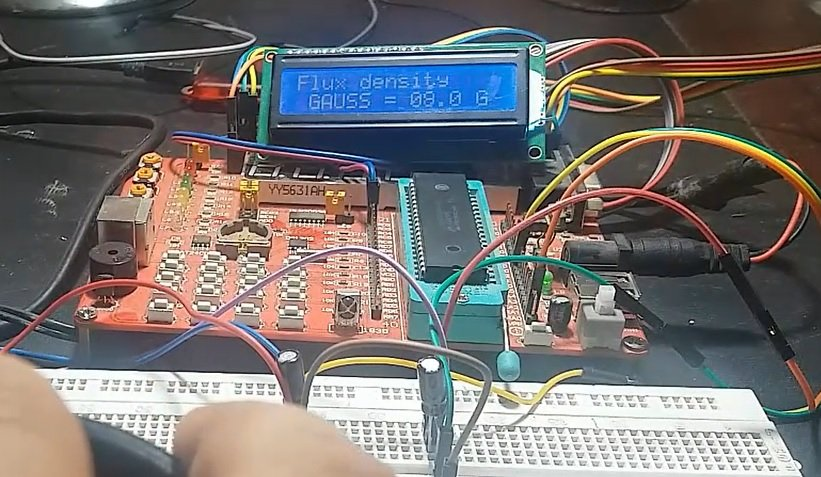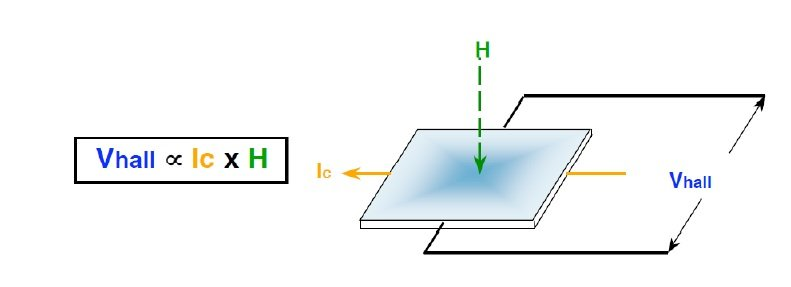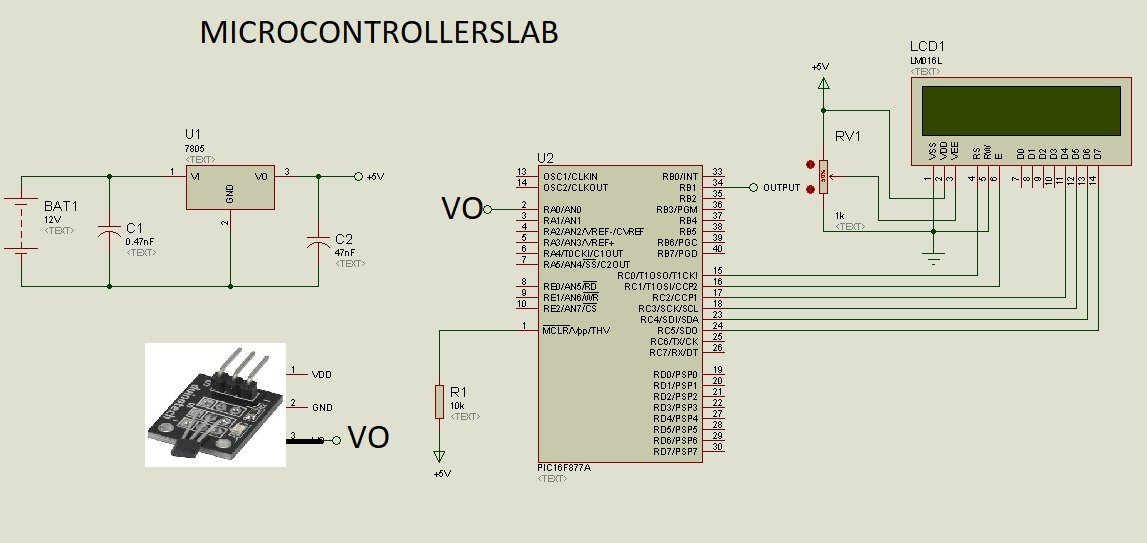# magnetic field strength measurement using pic microcontroller

magnetic field strength measurement using pic microcontroller, In this tutorial, you will learn about magnetic field strength measurement using pic microcontroller. magnetic strength measurement has many applications in physics and in electrical engineering projects.  In various industrial environment, we want to keep magnetic field under a specific limit and above this limit magnetic field may cause disturbance to electrical equipment’s. So magnetic field strength measurement project is useful for this kind of situation and we can easily magnetic strength measurement meter using a analog hall effect sensor and pic16F877A microcontroller.  Unit of magnetic field strength is Flux density and it is measured in Gauss. For more information on magnetic field check hereI have already posted articles using hall effect sensors, you may also like to read them:

So now lets start with the introduction to analog hall effect sensor.

### hall effect sensor for magnetic field strength measurement

Hall effect sensor produces output in the form of voltage. The magnitude of output voltage varies according to strength of magnetic field.  Greater the strength of magnetic field, higher will be the voltage the output of sensor. Hall effect sensors has many applications in position detection, speed measurement, magnetic field detection and magnetic field strength measurement.  There are two types of hall effect sensor modules available in marker. Linear hall effect sensor module and switch hall effect sensor module. We will be using linear hall effect sensor in tutorial along with two extra capacitors.  Working principle of hall effect sensor is shown in picture below:As shown above, voltage at the output of hall effect sensor is directly proportional to product to current and magnetic field strength. If we keep the current constant somehow, we can measure strength of magnetic field or flux density with the help of output voltage of the sensor. Because output voltage of sensor has direct relationship with magnetic field intensity.  The picture of linear hall effect sensor module,we are using shown below:This module has on board 10k ohm resistor and a light emitting diode. Fixed resistor will be used to keep constant current flowing through the hall effect sensor. With fixed current, flux density and voltage varies directly with each other. This module has three pins and details of each pin is given below:

• Ground pin
• 5 volt power supply pin
• Output voltage pin

### Magnetic field strength measurement using pic microcontroller

Circuit diagram for magnetic field strength measurement is given below. We just need to measure output voltage of sensor. The output voltage of sensor can be easily measured with built it analog to digital converter of pic16f877a microcontroller. Output of sensor is connected with analog channel zero of pic16f877a microcontroller. LCD is connected with PORTD of pic microcontroller which will be used to display flux density.  If you don’t know how to measure voltage and how to interface lcd with pic microcontroller check these articles:### Code

```// LCD module connections
sbit LCD_RS at RD2_bit;
sbit LCD_EN at RD3_bit;
sbit LCD_D4 at RD4_bit;
sbit LCD_D5 at RD5_bit;
sbit LCD_D6 at RD6_bit;
sbit LCD_D7 at RD7_bit;

sbit LCD_RS_Direction at TRISD2_bit;
sbit LCD_EN_Direction at TRISD3_bit;
sbit LCD_D4_Direction at TRISD4_bit;
sbit LCD_D5_Direction at TRISD5_bit;
sbit LCD_D6_Direction at TRISD6_bit;
sbit LCD_D7_Direction at TRISD7_bit;

char value[] = "GAUSS = 00.0 G";
char value1;
void main(void)
{
Lcd_Init(); // Initialize LCD
Lcd_Cmd(_LCD_CLEAR); // Clear display
Lcd_Cmd(_LCD_CURSOR_OFF); // Cursor off
Lcd_Out(1,1,"Flux density" ); // Write text in first row

while(1) { // Endless loop

lcd_out(2,2,"GAUSS = 00.0 G");
else
{